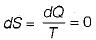Courses

# Test: Entropy - 2

## 10 Questions MCQ Test Topicwise Question Bank for Mechanical Engineering | Test: Entropy - 2

Description
This mock test of Test: Entropy - 2 for Mechanical Engineering helps you for every Mechanical Engineering entrance exam. This contains 10 Multiple Choice Questions for Mechanical Engineering Test: Entropy - 2 (mcq) to study with solutions a complete question bank. The solved questions answers in this Test: Entropy - 2 quiz give you a good mix of easy questions and tough questions. Mechanical Engineering students definitely take this Test: Entropy - 2 exercise for a better result in the exam. You can find other Test: Entropy - 2 extra questions, long questions & short questions for Mechanical Engineering on EduRev as well by searching above.
QUESTION: 1

### The relation, TdS = dU + PdV, is valid for

Solution:

TdS= dU + PdV
This equation holds good for any process reversible or irreversible, undergone by a closed system, since it is a relation among properties which are independent of the path.

QUESTION: 2

Solution:
QUESTION: 3

### In statistical thermodynamics, entropy is defined as 1. measure of irreversibility of a system 2. a universal property 3. degree of randomness 4. thermodynamic probability of disorderness Which of the above is/are correct

Solution:
QUESTION: 4

Energy tends over to gravitate to a lower degree of intensity so long as it undergoes no transformation.

Which law of thermodynamics is related to this statement

Solution:
QUESTION: 5

Consider the following statements:
In an irreversible process
1. entropy always increases
2. the sum of the entropy of all bodies taking part in a process always increases
3. once created, entropy can not be destroyed

Which among the above are correct?

Solution:

a) An irreversible process increases the entropy of the universe. Because entropy is a state function, the change in entropy of the system is the same, whether the process is reversible or irreversible.
b) The total entropy of a system either increases or remains constant; it never decreases. Entropy is zero in a reversible process; it increases in an irreversible process.
c) Once some entropy is created in a process , the universe has to carry the burden of that entropy forever .

QUESTION: 6

One kg of water at room temperature is brought into contact with a high temperature thermal reservoir. The entropy change of the universe is

Solution:
QUESTION: 7

For a thermodynamics cycle to be irreversible, it is necessary that

Solution: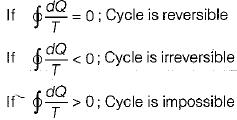Hence,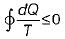⇒For cycle is possible This is known as Clausius inequality.

QUESTION: 8

Match List-I with List-ll and select the correct answer using the codes given below

List-I
A. Mechanical work
B.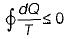C.  Zeroth law
D.  H - TS

List-II
1. Clausius-Clapeyron equation
2. Gibb’s equation
4. Concept of temperature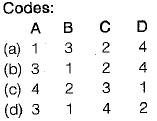Solution:

Gibbs function
G = H - TS
• Mechanical work is high grade energy
• Zeroth law gives the concept of temperature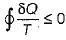is known as Clausius inequality

QUESTION: 9

Increase in entropy of a system represents

Solution:

Increase in entropy of a system leads to increase in randomness and degradation of energy.

QUESTION: 10

For reversible adiabatic process, change in entropy is

Solution: